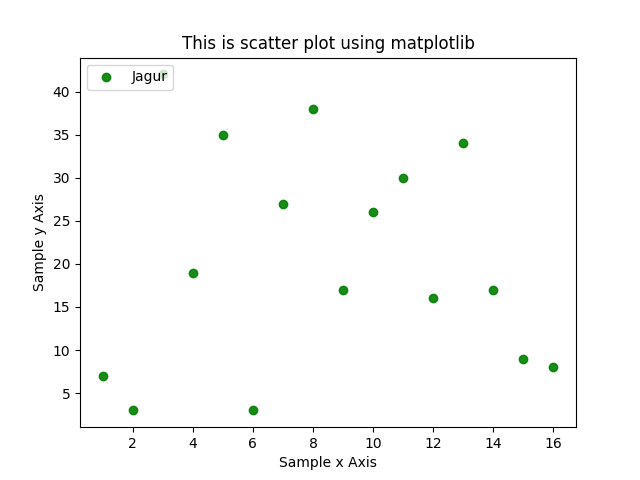# Scatter plots in Matplotlib – Data Visualization using Python

Scatter plots are one of the basic graphs used for data visualization. Scatter plot has following options, only first two are mandatory, rest all are optional.

`plt.scatter(x, y, s=None, c=None, marker=None, cmap=None, norm=None, vmin=None, vmax=None, alpha=None, linewidths=None, verts=None, edgecolors=None, hold=None, data=None, **kwargs)`

Here is simple program using scatter plot

Output of this program is as belowHere is a bit complex scatter plot using matplotlib